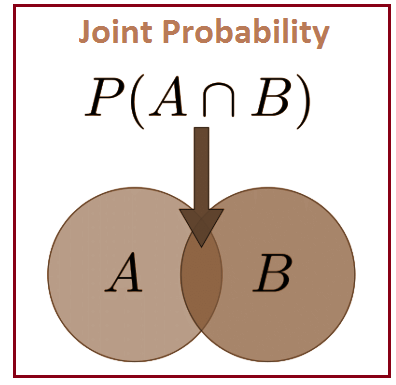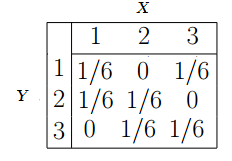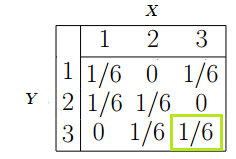# Joint Probability

Probability is a branch of mathematics which deals with the occurrence of a random event. In simple words it is the likelihood of a certain event. A statistical measure that calculates the likelihood of two events occurring together and at the same point in time is called Joint probability.

Let A and B be the two events, joint probability is the probability of event B occurring at the same time that event A occurs.

## Formula for Joint Probability

Notation to represent the joint probability can take a few different forms. The following formula represents the joint probability of events with intersection.

P (A⋂B)

where,

A, B= Two events

P(A and B),P(AB)=The joint probability of A and B

The symbol “∩” in a joint probability is called an intersection. The probability of event A and event B happening is the same thing as the point where A and B intersect. Hence, the joint probability is also called the intersection of two or more events. We can represent this relation using a Venn diagram as shown below.## Joint Probability Distribution

Let A, B, …., be the random variables which are defined on a probability space. The probability distribution that gives the probability that each of A, B, …. falls in any particular range or discrete set of values specified for that variable is defined as the joint probability distribution for A, B, ….. In the case of only two random variables, this is called a bivariate distribution, otherwise, it is a multivariate distribution.

The joint probability distribution can be expressed in different ways based on the nature of the variable. In case of discrete variables, we can represent a joint probability mass function. For continuous variables, it can be represented as a joint cumulative distribution function or in terms of a joint probability density function.

### Joint Probability Examples

Let us see some examples of how to find the joint probability with solutions.

Example: Find the probability that the number three will occur twice when two dice are rolled at the same time.

Solution:

Number of possible outcomes when a die is rolled = 6

i.e. {1, 2, 3, 4, 5, 6}

Let A be the event of occurring 3 on first die and B be the event of occurring 3 on the second die.

Both the dice have six possible outcomes, the probability of a three occurring on each die is 1/6.

P(A) =1/6

P(B )=1/6

P(A,B) = 1/6 x 1/6 = 1/36

### Joint Probability Table

A joint probability distribution represents a probability distribution for two or more random variables. Instead of events being labelled A and B, the condition is to use X and Y as given below.

f(x,y) = P(X = x, Y = y)

The main purpose of this is to look for a relationship between two variables. For example, the below table shows some probabilities for events X and Y happening at the same time:This table can be used to find the probabilities of events.

Example: Find the probability of X = 3 and Y = 3.

Solution: From the above table, identify the probability under X = 3 and Y= 3.

That is ⅙.